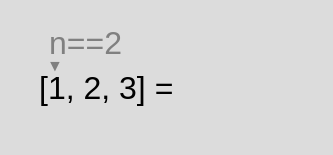## map

Creates a new array populated with the results of a provided function on every element.

``[1, 2, 3].map( n => n * 2 ); // [2, 4, 6]``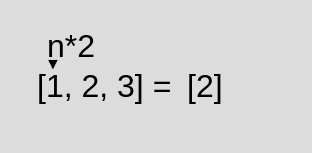## filter

Creates a Copy of a portion of a given array, filtered down to just the elements from the given array that pass the test, keep in mind that changes of your first array will affect your copy.

``[1,2,3].filter( n => n !== 2 ); // [1,3]``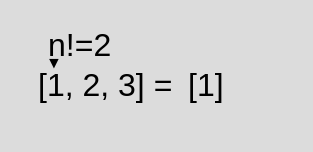## find

Stops and returns the first element in the provided array that satisfies the provided testing function, otherwise returns undefined.

``[1,2,3].find( n => n == 2 ) // 2``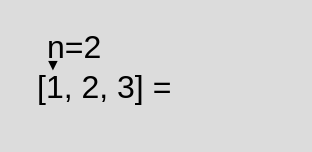## findIndex

Returns the index of the first element that satisfies the provided testing function. Otherwise -1 is returned.

``[1,2,3].findIndex( n => n == 2) // 1``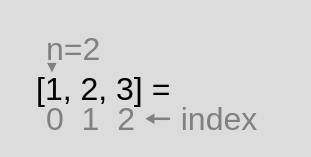## fill

Changes all elements in an array to a static value, from a start index (default 0) to an end index (default array.length). It returns the modified array.

``[1,2,3].fill('Txt', 1,2) // [1,'Txt', 3]``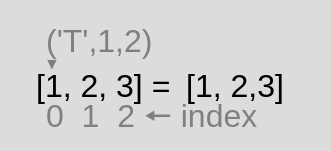## every

Tests all elements in the array. After finished returns a Boolean value

``[2,2,2].every( n => n == 2 ) // true``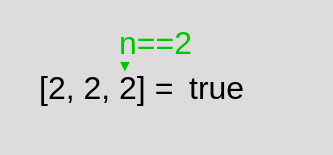## some

Tests whether at least one element in the array passes the test implemented by the provided function.

``[1,2,3].some( n => n == 2) // true``